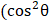# An elastic collision taken place between two smooth, rubber balls same radius as shown in fig. Initially, one ball is at rest and the other is moving with velocity. At maximum compressioni. ratio of potential to initial KE of the system is)/2 ii. ballis moving along directioniii. ballis moving along directionoriv. value ofcan not be used to finalise maximum compression velocities Evaluate the above statements and choose the correct option from the following: a) Statements i, ii are true and iii, iv are false b) Statements  i, ii are false and iii,iv are true c) All statements are true d) All statements are false

## Question ID - 100210 :- An elastic collision taken place between two smooth, rubber balls same radius as shown in fig. Initially, one ball is at rest and the other is moving with velocity. At maximum compressioni. ratio of potential to initial KE of the system is)/2 ii. ballis moving along directioniii. ballis moving along directionoriv. value ofcan not be used to finalise maximum compression velocities Evaluate the above statements and choose the correct option from the following: a) Statements i, ii are true and iii, iv are false b) Statements  i, ii are false and iii,iv are true c) All statements are true d) All statements are false

3537

(c)

Initial KEAt the time of maximum compression,Change inChange in KE= PEAt the time of max.Compression, impulse onis along directionand that onis in opposite direction. But the component of velocity ofin direction perpendicular tois unchanged

Next Question :
 A ball of massmoving with velocitymakes an oblique elastic collision with a stationary ball of mass 2The angle of divergence between the balls after  collision in ground frame, if the ball of massturns by an angle ofin centre of mass frame is a)b)c)d)